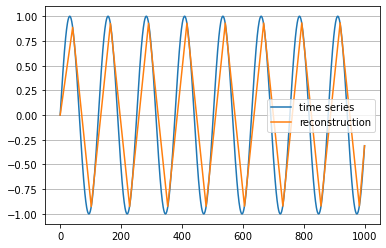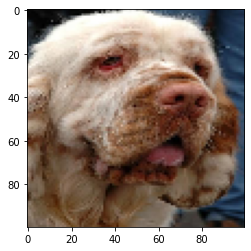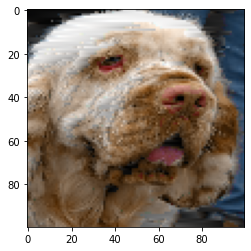# fABBA Release 0.9.9

An efficient aggregation method for the symbolic representation of temporal data

Keywords
dimensionality-reduction, knowledge-representation, machine-learning, time-series, time-series-analysis, time-series-classification, time-series-clustering, time-series-forecasting
BSD-3-Clause
Install
``` pip install fABBA==0.9.9 ```

# fABBA

### An efficient aggregation method for the symbolic representation of temporal data

fABBA is a fast and accurate symbolic representation method for temporal data. It is based on a polygonal chain approximation of the time series followed by an aggregation of the polygonal pieces into groups. The aggregation process is sped up by sorting the polygonal pieces and exploiting early termination conditions. In contrast to the ABBA method [S. Elsworth and S. Güttel, Data Mining and Knowledge Discovery, 34:1175-1200, 2020], fABBA avoids repeated within-cluster-sum-of-squares computations which reduces its computational complexity significantly. Furthermore, fABBA is fully tolerance-driven and does not require the number of time series symbols to be specified by the user.

## Install

To install the current release via PIP use:

``````pip install fABBA
``````

``````\$ git clone https://github.com/nla-group/fABBA.git
``````

## Examples

#### Compress and reconstruct a time series

The following example approximately transforms a time series into a symbolic string representation (`transform`) and then converts the string back into a numerical format (`inverse_transform`). fABBA essentially requires two parameters `tol` and `alpha`. The tolerance `tol` determines how closely the polygonal chain approximation follows the original time series. The parameter `alpha` controls how similar time series pieces need to be in order to be represented by the same symbol. A smaller `tol` means that more polygonal pieces are used and the polygonal chain approximation is more accurate; but on the other hand, it will increase the length of the string representation. A smaller `alpha` typically results in a larger number of symbols.

The choice of parameters depends on the application, but in practice, one often just wants the polygonal chain to mimic the key features in time series and not to approximate any noise. In this example the time series is a sine wave and the chosen parameters result in the symbolic representation `#\$!"!"!"!"!"!"!"%`. Note how the periodicity in the time series is nicely reflected in repetitions in its string representation.

```import numpy as np
import matplotlib.pyplot as plt
from fABBA import fabba_model

ts = [np.sin(0.05*i) for i in range(1000)]  # original time series
fabba = fabba_model(tol=0.1, alpha=0.1, sorting='2-norm', scl=1, verbose=0)

string = fabba.fit_transform(ts)            # string representation of the time series
print(string)                               # prints BbAaAaAaAaAaAaAaC

inverse_ts = fabba.inverse_transform(string, ts) # numerical time series reconstruction```

Plot the time series and its polygonal chain reconstruction:

```plt.plot(ts, label='time series', c='olive')
plt.plot(inverse_ts, label='reconstruction', c='darkblue')
plt.legend()
plt.grid(True, axis='y')
plt.show()```#### Adaptive polygonal chain approximation

Instead of using `transform` which combines the polygonal chain approximation of the time series and the symbolic conversion into one, both steps of fABBA can be performed independently. Here’s how to obtain the compression pieces and reconstruct time series by inversely transforming the pieces:

```import numpy as np
from fABBA import compress
from fABBA import inverse_compress
ts = [np.sin(0.05*i) for i in range(1000)]
pieces = compress(ts, tol=0.1)               # pieces is a list of the polygonal chain pieces
inverse_ts = inverse_compress(pieces, ts) # reconstruct polygonal chain from pieces```

Similarly, the digitization can be implemented after compression step as belows:

```from fABBA import digitize
from fABBA import inverse_digitize
string, parameters = digitize(pieces, alpha=0.1, sorting='2-norm', scl=1) # compression of the polygon
print(''.join(string))                                 # prints BbAaAaAaAaAaAaAaC

inverse_pieces = inverse_digitize(string, parameters)
inverse_ts = inverse_compress(inverse_pieces, ts)   # numerical time series reconstruction```

#### Alternative ABBA approach

We also provide other clustering based ABBA methods, it is easy to use with the support of scikit-learn tools. The user guidance is as follows

```import numpy as np
from sklearn.cluster import KMeans
from fABBA import ABBAbase

ts = [np.sin(0.05*i) for i in range(1000)]         # original time series
#  specifies 5 symbols using kmeans clustering
kmeans = KMeans(n_clusters=5, random_state=0, init='k-means++', verbose=0)
abba = ABBAbase(tol=0.1, scl=1, clustering=kmeans)
string = abba.fit_transform(ts)                    # string representation of the time series
print(string)                                      # prints BbAaAaAaAaAaAaAaC
inverse_ts = abba.inverse_transform(string)        # reconstruction```

#### Image compression

The following example shows how to apply fABBA to image data.

```import matplotlib.pyplot as plt
from fABBA import image_compress
from fABBA import image_decompress
from fABBA import fabba_model
from cv2 import resize
img_samples = load_images() # load test images
img = resize(img_samples, (100, 100)) # select the first image for test

fabba = fabba_model(tol=0.1, alpha=0.01, sorting='2-norm', scl=1, verbose=1)
string = image_compress(fabba, img)
inverse_img = image_decompress(fabba, string)```

Plot the original image:

```plt.imshow(img)
plt.show()```Plot the reconstructed image:

```plt.imshow(inverse_img)
plt.show()```## Experiments

The folder "exp" contains all code required to reproduce the experiments in the manuscript "An efficient aggregation method for the symbolic representation of temporal data".

Some of the experiments also require the UCR Archive 2018 datasets which can be downloaded from UCR Time Series Classification Archive.

There are a number of dependencies listed below. Most of these modules, except perhaps the final ones, are part of any standard Python installation. We list them for completeness:

`os, csv, time, pickle, numpy, warnings, matplotlib, math, collections, copy, sklearn, pandas, tqdm, tslearn`

Please ensure that these modules are available before running the codes. A `numpy` version newer than 1.19.0 is required.

It is necessary to compile the Cython files in the experiments folder (though this is already compiled in the main module, the experiments code is separated). To compile the Cython extension in "src" use:

``````\$ cd exp/src
\$ python3 setup.py build_ext --inplace
``````

or

``````\$ cd exp/src
\$ python setup.py build_ext --inplace
``````

## Others

We also provide C++ implementation for fABBA in the folder "cpp", it would be nice to give a shot!

Run example:

``````\$ git clone https://github.com/nla-group/fABBA.git
\$ cd fABBA/cpp
\$ g++ -o test runtime.cpp
\$ ./test
``````

## Software contributors

Xinye Chen (xinye.chen@manchester.ac.uk)

Stefan Güttel (stefan.guettel@manchester.ac.uk)

## Citation

If you use fABBA in a scientific publication, we would appreciate your citing:

```@techreport{CG22a,
title   = {An efficient aggregation method for the symbolic representation of temporal data},
author  = {Chen, Xinye and G\"{u}ttel, Stefan},
year    = {2022},
number  = {arXiv:2201.05697},
pages   = {23},
institution = {The University of Manchester},